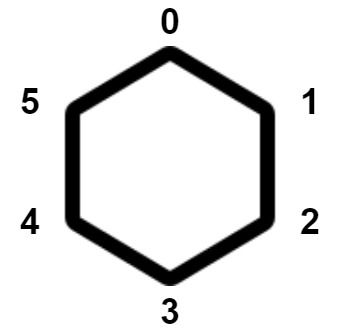##### Welcome to Subscribe On Youtube

Formatted question description: https://leetcode.ca/all/2550.html

# 2550. Count Collisions of Monkeys on a Polygon

## Description

There is a regular convex polygon with n vertices. The vertices are labeled from 0 to n - 1 in a clockwise direction, and each vertex has exactly one monkey. The following figure shows a convex polygon of 6 vertices.Each monkey moves simultaneously to a neighboring vertex. A neighboring vertex for a vertex i can be:

• the vertex (i + 1) % n in the clockwise direction, or
• the vertex (i - 1 + n) % n in the counter-clockwise direction.

A collision happens if at least two monkeys reside on the same vertex after the movement or intersect on an edge.

Return the number of ways the monkeys can move so that at least one collision happens. Since the answer may be very large, return it modulo 109 + 7.

Note that each monkey can only move once.

Example 1:

Input: n = 3
Output: 6
Explanation: There are 8 total possible movements.
Two ways such that they collide at some point are:
- Monkey 1 moves in a clockwise direction; monkey 2 moves in an anticlockwise direction; monkey 3 moves in a clockwise direction. Monkeys 1 and 2 collide.
- Monkey 1 moves in an anticlockwise direction; monkey 2 moves in an anticlockwise direction; monkey 3 moves in a clockwise direction. Monkeys 1 and 3 collide.
It can be shown 6 total movements result in a collision.


Example 2:

Input: n = 4
Output: 14
Explanation: It can be shown that there are 14 ways for the monkeys to collide.


Constraints:

• 3 <= n <= 109

## Solutions

• class Solution {
public int monkeyMove(int n) {
final int mod = (int) 1e9 + 7;
return (int) (qmi(2, n, mod) - 2 + mod) % mod;
}

public long qmi(long a, long k, long p) {
long res = 1;
while (k != 0) {
if ((k & 1) == 1) {
res = res * a % p;
}
k >>= 1;
a = a * a % p;
}
return res;
}
}

• class Solution {
public:
int monkeyMove(int n) {
const int mod = 1e9 + 7;
return (qmi(2, n, mod) - 2 + mod) % mod;
}

long qmi(long a, long k, long p) {
long res = 1;
while (k != 0) {
if ((k & 1) == 1) {
res = res * a % p;
}
k >>= 1;
a = a * a % p;
}
return res;
}
};

• class Solution:
def monkeyMove(self, n: int) -> int:
mod = 10**9 + 7
return (pow(2, n, mod) - 2) % mod


• func monkeyMove(n int) int {
const mod = 1e9 + 7
return (qmi(2, n, mod) - 2 + mod) % mod
}

func qmi(a, k, p int) int {
res := 1
for k != 0 {
if k&1 == 1 {
res = res * a % p
}
k >>= 1
a = a * a % p
}
return res
}

• function monkeyMove(n: number): number {
const mod = BigInt(10 ** 9 + 7);
return Number((qmi(2n, n, mod) - 2n + mod) % mod);
}

function qmi(a: bigint, k: number, p: bigint): bigint {
let res = 1n;
while (k) {
if ((k & 1) === 1) {
res = (res * a) % p;
}
k >>= 1;
a = (a * a) % p;
}
return res;
}